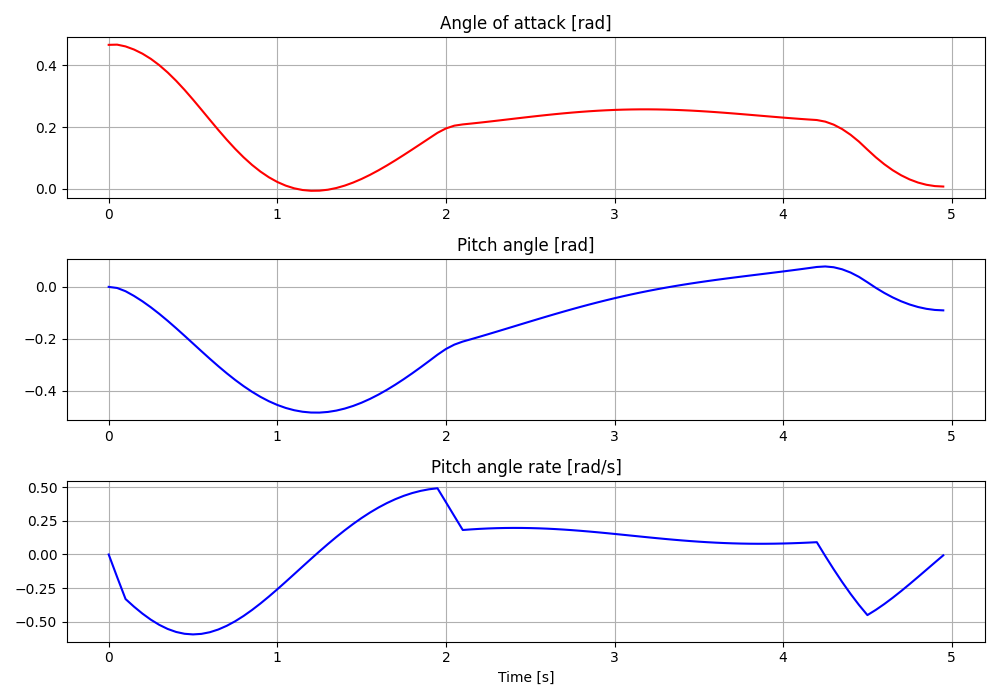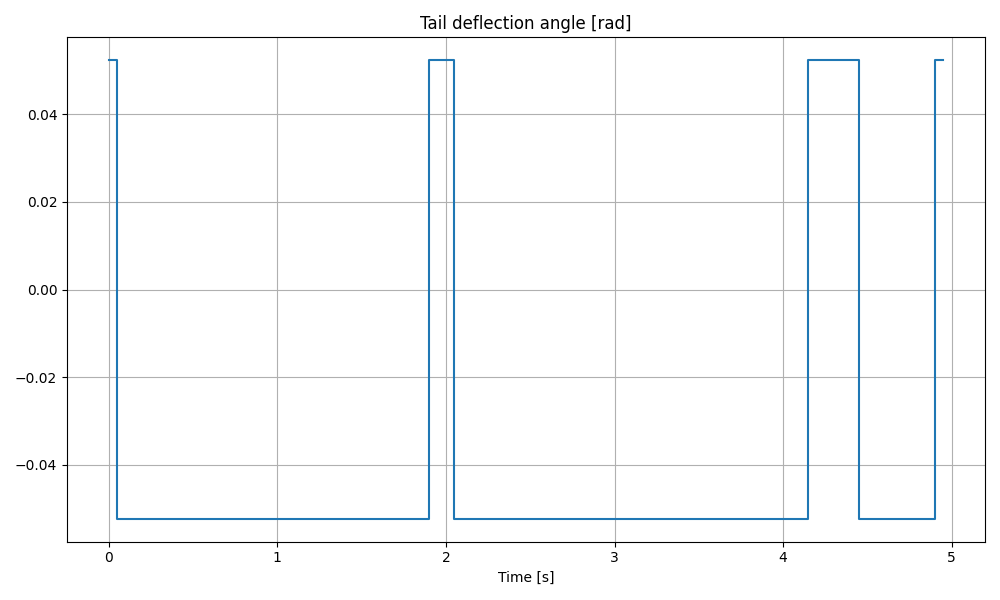# 8.10. Mixed-integer nonlinear solver: F8 Crusader aircraft¶

In this example we illustrate the simplicity of the high-level user interface on a mixed-integer nonlinear program. In particular, we use an F8 Crusader aircraft model described by a set of ordinary differential equations (ODEs):

$\begin{split}\dot{x}_0 = & -0.877 x_0 + x_2 - 0.088 x_0 x_2 + 0.47 x_0^{2} - 0.019 x_1^{2} - x_0^{2} x_2 + 3.846 x_0^{3} \\ & -0.215 w + 0.28 x_0^2w + 0.47 x_0w^{2} + 0.63 w^{3} \\ \dot{x}_1 = & x_2 \\ \dot{x}_2 = & -0.4208 x_0 - 0.396 x_2 - 0.47 x_0^{2} - 3.564 x_0^{3} - 20.967 w \\ & + 6.265 x_0^{2}w + 46 x_0w^{2} + 61.4 w^{3}\end{split}$

The model is taken from [GarJor77] and consists of three differential states: $$x_0$$ the angle of attack in radians, $$x_1$$ the pitch angle in radians and $$x_2$$ the pitch angle rate in radians per second. There is one control input $$w$$, the tail deflection angle in radians. The input is the discrete component of the model, since it can take values within the discrete set $$\{-0.05236, 0.05236\}$$. This makes the solution process more complicated in comparison to a nonlinear program, as the different combinations of inputs have to be checked over the control horizon.

The trajectory of the aircraft is to be computed by solving a mixed-integer nonlinear program (MINLP). First, we define the stage variable $$z$$ by stacking the input and differential state variables:

$z = [w, x_0, x_1, x_2]^\top$

You can download the Matlab code of this example to try it out for yourself by clicking here.

## 8.10.1. Defining the problem data¶

### 8.10.1.1. Objective¶

Our goal is to minimize the distance of the final state to the origin, which can be translated in the following cost function on the final stage variable:

$f(z) = 150 x_0^{2} + 5 x_1^{2} + 5 x_2^{2}$

The terminal cost function is coded in MATLAB as the following function:

model.objectiveN = @(z) 150 * z(2)^2 + 5 * z(3)^2 + 5 * z(4)^2;


Moreover, control inputs are penalized at every stage via the following stage cost function:

model.objective = @(z) 0.1 * z(1)^2;


### 8.10.1.2. Equality constraints¶

In this example, the only equality constraints are related to the dynamics. They are provided to FORCES Pro in continuous form. The discretization is then computed internally by the FORCES Pro integrators.

In the code snippet below, it is important to notice that the control input $$w$$ is replaced with $$u$$ such that

$w~~\hat{=}~~0.05236~\cdot~(2 u - 1)$

If $$w$$ has values within $$\{-0.05236, 0.05236\}$$, then $$u$$ lies within the binary set $$\{0, 1\}$$.

wa = 0.05236;
wa2 = wa^2;
wa3 = wa^3;
continuous_dynamics = @(x, u) [-0.877 * x(1) + x(3) - 0.088 * x(1) * x(3)...
+ 0.47 * x(1) * x(1) - 0.019 * x(2) * x(2)...
- x(1) * x(1) * x(3)...
+ 3.846 * x(1) * x(1) * x(1)...
- 0.215 * wa * (2 * u(1) - 1)...
+ 0.28 * x(1) * x(1) * wa * (2 * u(1) - 1)...
+ 0.47*x(1)*wa2*(2*u(1)-1)*(2*u(1)-1)...
+ 0.63*wa3*(2*u(1)-1)*(2*u(1)-1)*(2*u(1)-1);
x(3);
-4.208*x(1) - 0.396 * x(3) - 0.47 * x(1)*x(1)...
- 3.564 * x(1) * x(1) * x(1)...
- 20.967 * wa * (2 * u(1) - 1)...
+ 6.265 * x(1) * x(1) * wa * (2 * u(1) -1 )...
+ 46.0 * x(1)*wa2*(2*u(1)-1)*(2*u(1)-1)...
+ 61.4*wa3*(2*u(1)-1)*(2*u(1)-1)*(2*u(1)-1)];
model.continuous_dynamics = continuous_dynamics;
model.E = [zeros(3, 1), eye(3)];


### 8.10.1.3. Inequality constraints¶

The maneuver is subjected to a set of constraints, involving only the simple bounds:

### 8.10.1.4. Initial and final conditions¶

The goal of the maneuver is to steer the aircraft from an initial condition with nose pointing upwards

$(0.4655, 0, 0)^{T}$

to the origin.

## 8.10.2. Defining the MPC problem¶

With the above de fined MALTAB functions for objective and equality constraints, we can completely define the MINLP formulation in the next code snippet. For this example, the number of stages has been set to $$N = 100$$.

In the code snippet below, it is important to notice that the lower and upper bounds are declared as parametric before generating the solver. This needs to be done for generating mixed-integer NLP solvers. Lower and upper bounds are meant to be provided at run-time.

%% Problem dimension
nx = 3;
nu = 1;
nz = nx + nu;
model.N = 100;
model.nvar = nz;
model.neq = nx;

%% Indices of initial state in stage variable
model.xinitidx = nu+1:model.nvar;

%% Lower and upper bound need to be set as parametric for generating an MINLP solver
model.lb = [];
model.ub = [];
model.lbidx{1} = 1 : nu;
model.ubidx{1} = 1 : nu;
for i = 2 : model.N
model.lbidx{i} = 1 : model.nvar;
model.ubidx{i} = 1 : model.nvar;
end

%% Dynamics
wa = 0.05236;
wa2 = wa^2;
wa3 = wa^3;
continuous_dynamics = @(x, u) [-0.877 * x(1) + x(3) - 0.088 * x(1) * x(3)...
+ 0.47 * x(1) * x(1) - 0.019 * x(2) * x(2)...
- x(1) * x(1) * x(3)...
+ 3.846 * x(1) * x(1) * x(1)...
- 0.215 * wa * (2 * u(1) - 1)...
+ 0.28 * x(1) * x(1) * wa * (2 * u(1) - 1)...
+ 0.47 *x(1)*wa2*(2*u(1)-1)*(2*u(1)-1)...
+ 0.63*wa3*(2*u(1)-1)*(2*u(1)-1)*(2*u(1)-1);
x(3);
-4.208 * x(1) - 0.396 * x(3)...
- 0.47 * x(1) * x(1)...
- 3.564 * x(1) * x(1) * x(1)...
- 20.967 * wa * (2 * u(1) - 1)...
+ 6.265*x(1)*x(1)*wa*(2*u(1)-1)...
+ 46.0*x(1)*wa2*(2*u(1)-1)*(2*u(1)-1)...
+ 61.4*wa3*(2*u(1)-1)*(2*u(1)-1)*(2*u(1)-1)];
model.continuous_dynamics = continuous_dynamics;
model.E = [zeros(nx, nu), eye(nx)];

%% Objective
mode.objective =  @(z) 0.1 * z(nu)^2;
model.objectiveN = @(z) 150 * z(nu+1)^2...
+ 5 * z(nu+2)^2...
+ 5 * z(nu+3)^2;

%% Indices of integer variables within every stage
for s = 1:model.N
model.intidx{s} = ;
end


## 8.10.3. Generating an MINLP solver¶

We have now populated model with the necessary fields to generate a mixed-integer solver for our problem. Now we set some options for our solver and then use the function FORCES_NLP to generate a solver for the problem defined by model with the initial state and the lower and upper bounds as a parameters:

%% Set code-generation options
codeoptions = getOptions('F8aircraft');
codeoptions.printlevel = 1;
codeoptions.misra2012_check = 1;
codeoptions.maxit = 2000;
codeoptions.timing = 0;
codeoptions.nlp.integrator.type = 'IRK2';
codeoptions.nlp.integrator.Ts = 0.05;
codeoptions.nlp.integrator.nodes = 20;

%% Generate MINLP solver
FORCES_NLP(model, codeoptions);


In the code snippet above, we have set some integrator options, since the continuous-time dynamics has been provided in the model. The branch-and-bound search can be run on several threads in parallel by setting the run-time parameter numThreadsBnB equal to the number of threads to be used. The default value is 1. Moreover, the maximum number of threads for the branch-and-bound search can be set via the option max_num_threads. By default, max_num_threads = 4.

## 8.10.4. Calling the generated MINLP solver¶

Once all parameters have been populated, the MEX interface of the solver can be used to invoke it:

%% Set run-time parameters
problem.(sprintf('lb%02d', 1)) = 0;
problem.(sprintf('ub%02d', 1)) = 1;
for s = 2:99
problem.(sprintf('lb%02d', s)) = [0, -1e1 * ones(1, 3)]';
problem.(sprintf('ub%02d', s)) = [1, 1e1 * ones(1, 3)]';
end
problem.(sprintf('lb%02d', 100)) = [0, -1e1 * ones(1, 3)]';
problem.(sprintf('ub%02d', Nstages)) = [1, 1e1 * ones(1, 3)]';

problem.x0 = repmat([0; zeros(3, 1)], 100, 1);
problem.xinit = zeros(3, 1);
problem.xinit(1) = 0.4655;

%% Call MINLP solver
[sol, exitflag, info] = F8aircraft(problem);


## 8.10.5. Providing an initial guess at run-time¶

In order to provide an guess for the incumbent, the following code-generation options need to be enabled:

codeoptions.minlp.int_guess = 1;
codeoptions.minlp.round_root = 0; % Default value is 1
codeoptions.minlp.int_guess_stage_vars = ; % An integer guess is provided for variable 1 at every stage


Then the incumbent guess can be set at run-time via

for s = 1:Nstages
problem.(sprintf('int_guess%03d', s)) = ;
end
for s = 1:2
problem.(sprintf('int_guess%03d', s)) = ;
end
problem.(sprintf('int_guess%03d', 39)) = ;
for s = 41:42
problem.(sprintf('int_guess%03d', s)) = ;
end
for s = 85:90
problem.(sprintf('int_guess%03d', s)) = ;
end


## 8.10.6. Changing the parallelization strategy at run-time¶

When running the MINLP solver on several threads with numThreadsBnB >= 1, the parallelization strategy can be changed via

problem.parallelStrategy = 0; % 0 (one shared priority queue, default), 1 (one priority queue per thread)


## 8.10.7. Results¶

The control objective is to drive the angle of attack as close as possible to zero within a five seconds time frame. The control input is the tail deflection angle, which can take values with the set $$\{-0.05236, 0.05236\}$$ and the initial state is $$(0.4655, 0, 0)^{T}$$, where the first component is the angle of attack, the second component is the pitch angle and the third component is the pitch angle rate.

The angle of attack computed by FORCES Pro MINLP solver running on one thread is shown in Figure Figure 8.46 and the input sequence is in Figure Figure 8.47. One can notice the bang-bang behaviour of the solution. When running on three threads the FORCES Pro MINLP solver provides a solution with lower final primal objective. Results are shown on Figures Figure 8.48 and Figure 8.49.Figure 8.46 Aircraft’s angle of attack over time computed with one thread.Figure 8.47 Aircraft’s tail deflection angle over time with one thread.Figure 8.48 Aircraft’s angle of attack over time computed with three threads.Figure 8.49 Aircraft’s tail deflection angle over time with three threads.

GarJor77

Garrard, W.L.; Jordan, J.M..: Design of Nonlinear Automatic Control Systems. In: Automatica 1977, vol. 13, 497-505.